# 8086 Instruction Format

## 8086 Instruction Format:

The 8086 Instruction 8086 Instruction Format vary from 1 to 6 bytes in length. Fig. 6.8 shows the instruction formats for 1 to 6 bytes instructions. As shown in the Fig. 6.8, displacements and operands may be either 8-bits or 16-bits long depending on the instruction. The opcode and the addressing mode is specified using first two bytes of an instruction.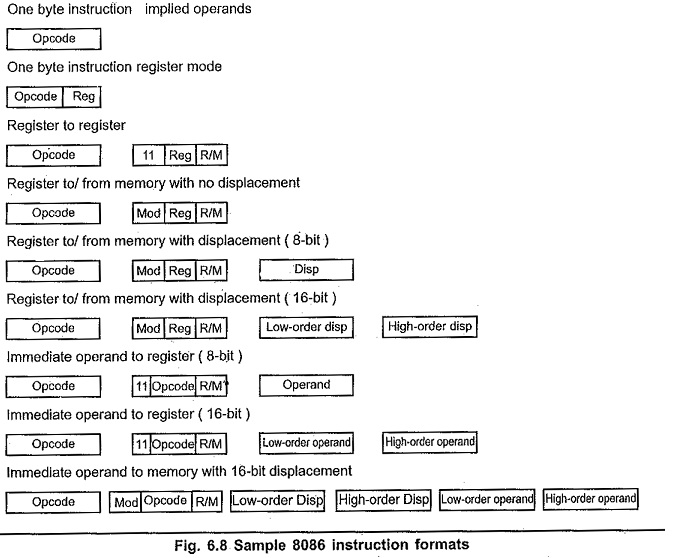The opcode/addressing mode byte(s) may be followed by :

• Two byte EA (For direct addressing only).
• One or two byte displacement
• One or two byte immediate operand
• One or two byte displacement followed by a one or two byte immediate operand
• Two byte displacement and a two byte segment address (for direct intersegment addressing only).

Most of the opcodes in 8086 has a special 1-bit indicates. They are :

W-bit : Some instructions of 8086 can operate on byte or a word. The W-bit in the opcode of such instruction specify whether instruction is a byte instruction (W = 0) or a word instruction (W = 1).

D-bit : The D-bit in the opcode of the instruction indicates that the register specified within the instruction is a source register (D = 0) or destination register (D =1).

S-bit : An 8-bit 2’s complement number can be extended to a 16-bit 2’s complement number by making all of the bits in the higher-order byte equal the most significant bit in the low order byte. This is known as sign extension. The S-bit along with the W-bit indicate :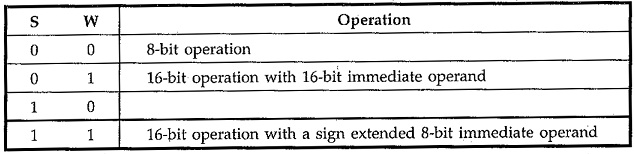V-bit : V-bit decides the number of shifts for rotate and shift instructions. If V = 0, then count = 1; if V = 1, the count is in CL register. For example, if V = 1 and CL = 2 then shift or rotate instruction shifts or rotates 2-bits

Z-bit : It is used for string primitives such as REP for comparison with ZF Flag. (Refer Appendix A for instruction formats)

As seen from the Fig. 6.8 if an instruction has two opcode/addressing mode bytes, then the second byte is of one of the following two forms .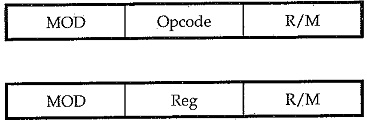where Mod, Reg and R/M fields specify operand as described in the following tables.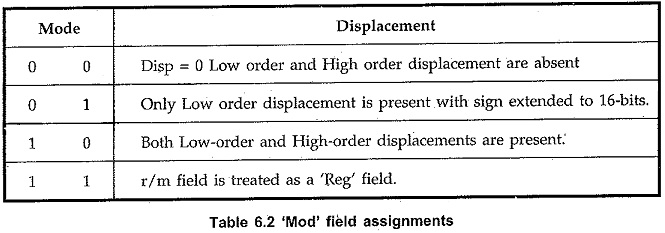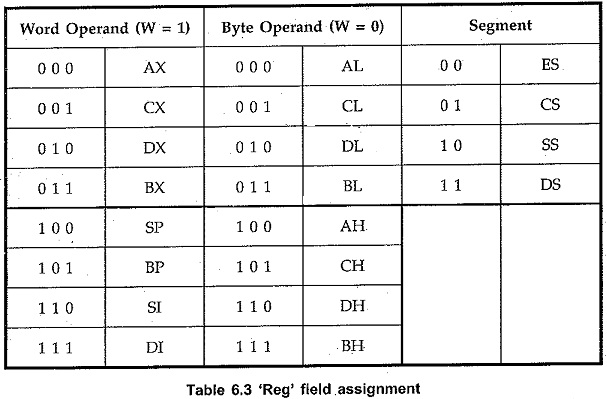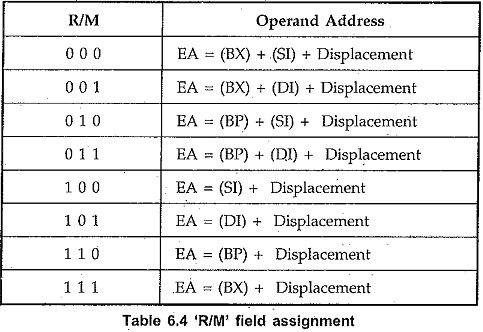Updated: August 8, 2018 — 8:34 pm